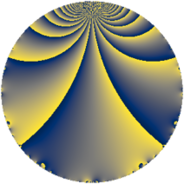# Properties

 Label 363.3.bLevel $363$ Weight $3$ Character orbit 363.b Rep. character $\chi_{363}(122,\cdot)$ Character field $\Q$ Dimension $64$ Newform subspaces $14$ Sturm bound $132$ Trace bound $7$

# Related objects

## Defining parameters

 Level: $$N$$ $$=$$ $$363 = 3 \cdot 11^{2}$$ Weight: $$k$$ $$=$$ $$3$$ Character orbit: $$[\chi]$$ $$=$$ 363.b (of order $$2$$ and degree $$1$$) Character conductor: $$\operatorname{cond}(\chi)$$ $$=$$ $$3$$ Character field: $$\Q$$ Newform subspaces: $$14$$ Sturm bound: $$132$$ Trace bound: $$7$$ Distinguishing $$T_p$$: $$2$$, $$5$$, $$7$$

## Dimensions

The following table gives the dimensions of various subspaces of $$M_{3}(363, [\chi])$$.

Total New Old
Modular forms 100 82 18
Cusp forms 76 64 12
Eisenstein series 24 18 6

## Trace form

 $$64 q - 108 q^{4} + 2 q^{6} + 12 q^{7} - 16 q^{9} + O(q^{10})$$ $$64 q - 108 q^{4} + 2 q^{6} + 12 q^{7} - 16 q^{9} - 36 q^{10} + 10 q^{12} + 10 q^{15} + 172 q^{16} - 38 q^{18} + 48 q^{19} + 86 q^{21} - 24 q^{24} - 204 q^{25} - 66 q^{27} - 48 q^{28} - 142 q^{30} + 36 q^{31} - 36 q^{34} + 262 q^{36} - 28 q^{37} + 32 q^{39} + 168 q^{40} + 108 q^{42} - 180 q^{43} - 34 q^{45} + 132 q^{46} + 10 q^{48} + 132 q^{49} - 214 q^{51} + 192 q^{52} + 176 q^{54} - 108 q^{57} - 532 q^{58} - 352 q^{60} - 14 q^{63} - 168 q^{64} - 28 q^{67} + 358 q^{69} + 324 q^{70} - 72 q^{72} + 432 q^{73} + 170 q^{75} - 264 q^{76} + 232 q^{78} + 156 q^{79} - 208 q^{81} + 168 q^{82} - 416 q^{84} - 108 q^{85} + 252 q^{87} - 410 q^{90} - 236 q^{91} - 54 q^{93} + 312 q^{94} - 272 q^{96} - 484 q^{97} + O(q^{100})$$

## Decomposition of $$S_{3}^{\mathrm{new}}(363, [\chi])$$ into newform subspaces

Label Dim $A$ Field CM Traces $q$-expansion
$a_{2}$ $a_{3}$ $a_{5}$ $a_{7}$
363.3.b.a $1$ $9.891$ $$\Q$$ $$\Q(\sqrt{-3})$$ $$0$$ $$-3$$ $$0$$ $$-11$$ $$q-3q^{3}+4q^{4}-11q^{7}+9q^{9}-12q^{12}+\cdots$$
363.3.b.b $1$ $9.891$ $$\Q$$ $$\Q(\sqrt{-3})$$ $$0$$ $$-3$$ $$0$$ $$11$$ $$q-3q^{3}+4q^{4}+11q^{7}+9q^{9}-12q^{12}+\cdots$$
363.3.b.c $2$ $9.891$ $$\Q(\sqrt{-11})$$ $$\Q(\sqrt{-11})$$ $$0$$ $$5$$ $$0$$ $$0$$ $$q+(3-\beta )q^{3}+4q^{4}+(-3+6\beta )q^{5}+\cdots$$
363.3.b.d $2$ $9.891$ $$\Q(\sqrt{-11})$$ None $$0$$ $$6$$ $$0$$ $$16$$ $$q-\beta q^{2}+3q^{3}-7q^{4}-2\beta q^{5}-3\beta q^{6}+\cdots$$
363.3.b.e $2$ $9.891$ $$\Q(\sqrt{3})$$ $$\Q(\sqrt{-3})$$ $$0$$ $$6$$ $$0$$ $$0$$ $$q+3q^{3}+4q^{4}-5\beta q^{7}+9q^{9}+12q^{12}+\cdots$$
363.3.b.f $4$ $9.891$ $$\Q(i, \sqrt{5})$$ None $$0$$ $$-8$$ $$0$$ $$-22$$ $$q-\beta _{3}q^{2}+(-2-2\beta _{1}-\beta _{3})q^{3}+3q^{4}+\cdots$$
363.3.b.g $4$ $9.891$ $$\Q(i, \sqrt{5})$$ None $$0$$ $$-8$$ $$0$$ $$22$$ $$q+\beta _{3}q^{2}+(-2-2\beta _{1}-\beta _{3})q^{3}+3q^{4}+\cdots$$
363.3.b.h $4$ $9.891$ $$\Q(\sqrt{-3}, \sqrt{-11})$$ None $$0$$ $$-5$$ $$0$$ $$-4$$ $$q+(-\beta _{1}-\beta _{2}+\beta _{3})q^{2}+(-1+\beta _{2}+\cdots)q^{3}+\cdots$$
363.3.b.i $4$ $9.891$ $$\Q(\sqrt{-2}, \sqrt{-7})$$ None $$0$$ $$-4$$ $$0$$ $$0$$ $$q+\beta _{2}q^{2}+(-1+\beta _{3})q^{3}-3q^{4}+\beta _{3}q^{5}+\cdots$$
363.3.b.j $6$ $9.891$ $$\mathbb{Q}[x]/(x^{6} + \cdots)$$ None $$0$$ $$4$$ $$0$$ $$0$$ $$q+\beta _{1}q^{2}+(1-\beta _{3})q^{3}+(-3-\beta _{1}+\beta _{2}+\cdots)q^{4}+\cdots$$
363.3.b.k $6$ $9.891$ $$\mathbb{Q}[x]/(x^{6} + \cdots)$$ None $$0$$ $$4$$ $$0$$ $$0$$ $$q+\beta _{1}q^{2}+(1-\beta _{2})q^{3}+(-3+\beta _{1}-\beta _{2}+\cdots)q^{4}+\cdots$$
363.3.b.l $8$ $9.891$ $$\mathbb{Q}[x]/(x^{8} + \cdots)$$ None $$0$$ $$5$$ $$0$$ $$-28$$ $$q+\beta _{1}q^{2}+(-\beta _{2}+\beta _{3}-\beta _{5}+\beta _{6})q^{3}+\cdots$$
363.3.b.m $8$ $9.891$ $$\mathbb{Q}[x]/(x^{8} + \cdots)$$ None $$0$$ $$5$$ $$0$$ $$28$$ $$q+\beta _{1}q^{2}+(-\beta _{2}+\beta _{4}+\beta _{5})q^{3}+(-4+\cdots)q^{4}+\cdots$$
363.3.b.n $12$ $9.891$ $$\mathbb{Q}[x]/(x^{12} - \cdots)$$ None $$0$$ $$-4$$ $$0$$ $$0$$ $$q-\beta _{1}q^{2}+\beta _{4}q^{3}+(-4+\beta _{2}-\beta _{4}+\cdots)q^{4}+\cdots$$

## Decomposition of $$S_{3}^{\mathrm{old}}(363, [\chi])$$ into lower level spaces

$$S_{3}^{\mathrm{old}}(363, [\chi]) \cong$$ $$S_{3}^{\mathrm{new}}(33, [\chi])$$$$^{\oplus 2}$$